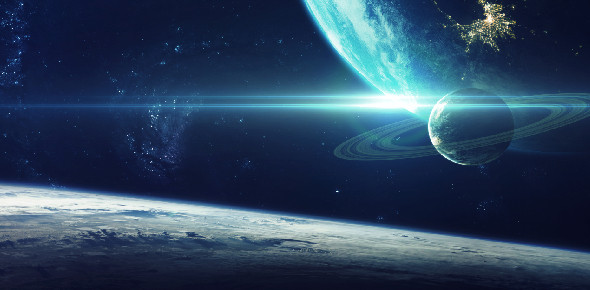# Space Quiz

10 QuestionsSettings​

Related Topics
• 1.
• A.

Gravity

• B.

Planets

• C.

Stars

• D.

None of the above

• E.

By Mahika

• 2.
• A.

Gas

• B.

Another star

• C.

Supernova

• D.

None of the above

• E.

By Mahika

• 3.
• A.

To old

• B.

No more fuel

• C.

For no reason

• D.

None of the above

• E.

By Mahika

• 4.
• A.

Nothing

• B.

Planets die

• C.

Stars fall

• D.

None of the above

• E.

By Mahika

• 5.
• A.

Red dwarf star

• B.

Neutron star

• C.

Porto star

• D.

None of the above

• E.

By James

• 6.
• A.

Red giant

• B.

Blue star

• C.

Red star

• D.

None of the above

• E.

By James

• 7.
• A.

As much as there are grains of star on earth

• B.

More than all the grains of sand on earth

• C.

As much as all over earth

• D.

None of the above

• E.

By James

• 8.
• A.

They don't move

• B.

A mile a month

• C.

They move thousands of miles per second

• D.

None of the above

• E.

By Eigo

• 9.
• A.

All the same

• B.

Some galaxies are big some are small

• C.

Galaxies are always changing sizes

• D.

None of the above

• E.

By Eigo

• 10.
How long does it take for Andromeda to reach us?
• A.

1 million years

• B.

3 million years

• C.

2 million years

• D.

None of the above

• E.

By Eigo